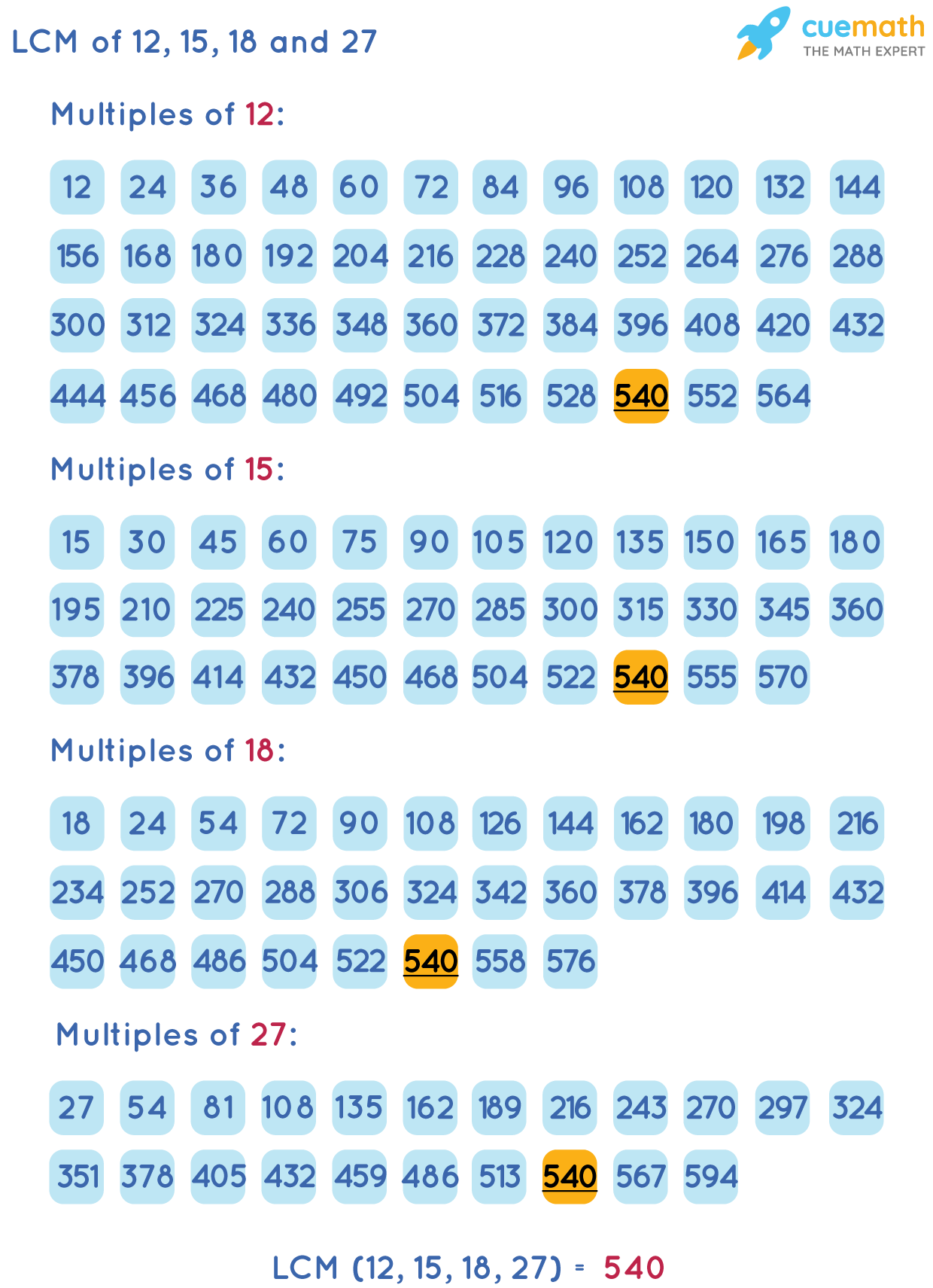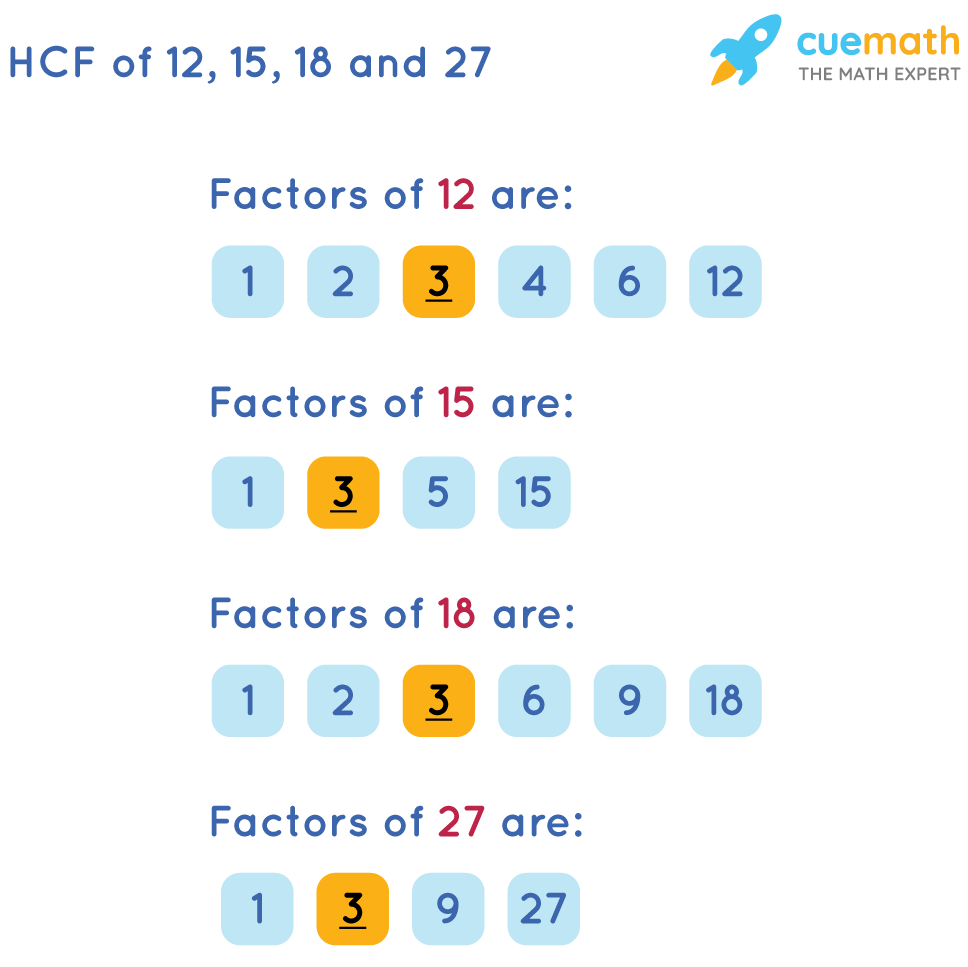# Find The HCF and LCM of 12 15 18 and 27

## Question: Find the HCF and LCM of 12 15 18 and 27?

To find the Least Common Multiple (LCM) of a, b, c and d, we need the least number which is exactly divisible by all the numbers a, b, c, and d without leaving any remainder.

## Answer: LCM of 12 15 18, and 27 is 504, and the HCF of 12, 15, 18, and 27 is 3

The Highest Common Factor (HCF) is the largest possible number which divides all the numbers exactly without leaving any remainder.

## Explanation:

We are using the Listing Method to find the LCM of 12, 15, 18, and 27

We will list the first few multiples of 12, 15, 18, and 27 and determine the common multiples.

The least among the common multiples is the LCM of 12, 15, 18, and 27Here, 540 is the least common multiple of 12, 15, 18, and 27. So, the LCM of 12, 15, 18, and 27 is 540.

Now we are using the methods of Listing Common Factors to find the HCF of 12, 15, 18, and 27

The factors of 12, 15, 18, and 27 are shown below.The common factor of 12, 15, 18, and 27 are 1 and 3

HCF is the product of the factors that are common to each of the given numbers.

So, HCF of 12, 15, 18, and 27 is 3